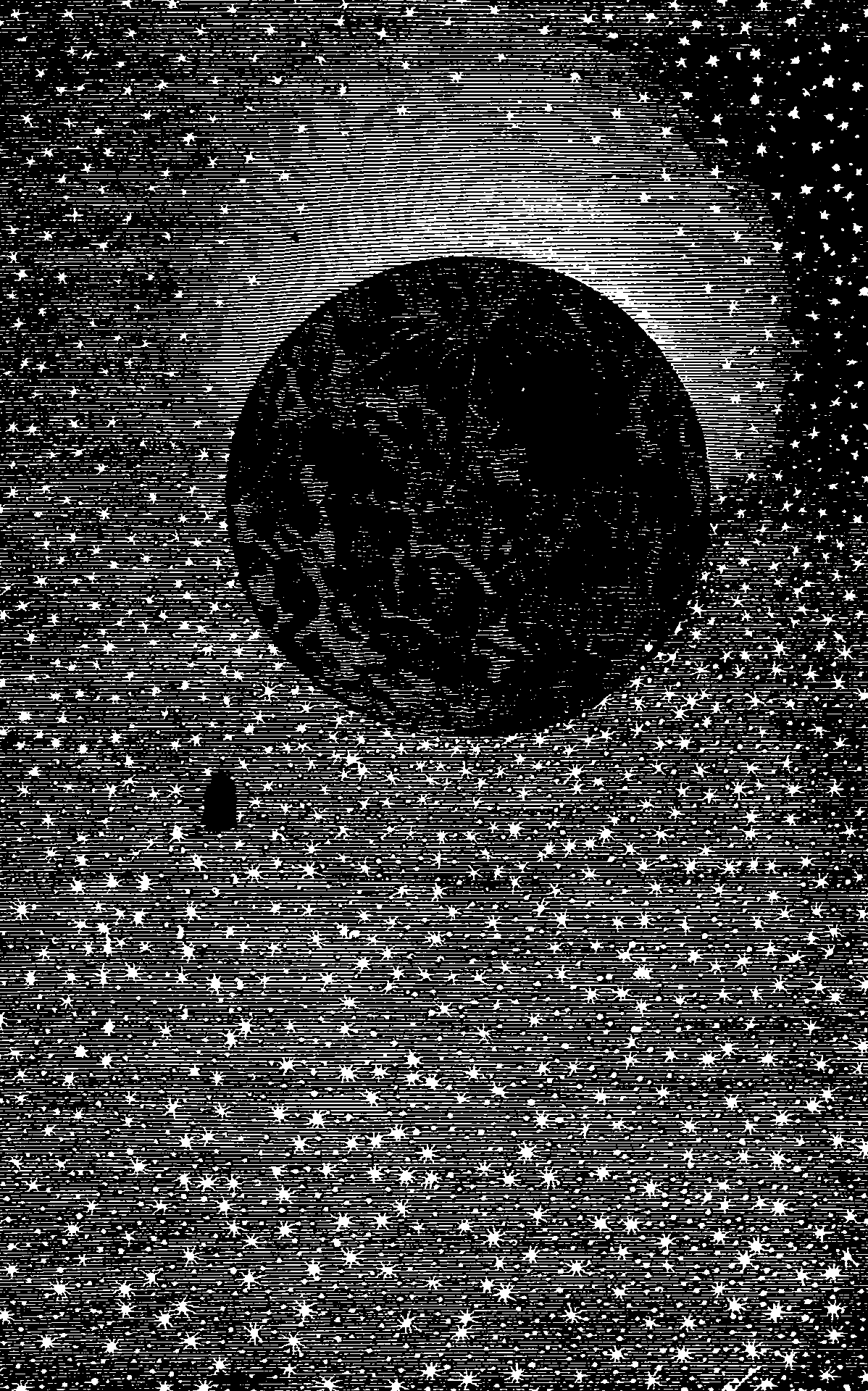# Potential theory in probability

## Something about harmonic functions or whateverPlaceholder.

A theory about something to do with expectations of harmonic functions. Not the same thing as the potentials that arise as an alternative term to factor in factor graphs; that is different.

I am unfamiliar with potential theory as a thing in itself, but it arises in Markov processes, exp Lévy processes and Markov bridge processes.

The origins of potential theory can be traced to the physical problem of reconstructing a repartition of electric charges inside a planar or a spatial domain, given the measurement of the electrical field created on the boundary of this domain.

Privault (2008):

In mathematical analytic terms this amounts to representing the values of a function $$h$$ inside a domain given the data of the values of $$h$$ on the boundary of the domain. In the simplest case of a domain empty of electric charges, the problem can be formulated as that of finding a harmonic function $$h$$ on $$E$$ (roughly speaking, a function with vanishing Laplacian, see $$\S 2.2$$ below), given its values prescribed by a function $$f$$ on the boundary $$\partial E$$, i.e. as the Dirichlet problem: $\begin{cases}\Delta h(y)=0, & y \in E, \\ h(y)=f(y), & y \in \partial E\end{cases}$ Close connections between the notion of potential and the Markov property have been observed at early stages of the development of the theory, see e.g. Doob (2001) and references therein. Thus a number of potential theoretic problems have a probabilistic interpretation or can be solved by probabilistic methods.

### No comments yet. Why not leave one?

GitHub-flavored Markdown & a sane subset of HTML is supported.